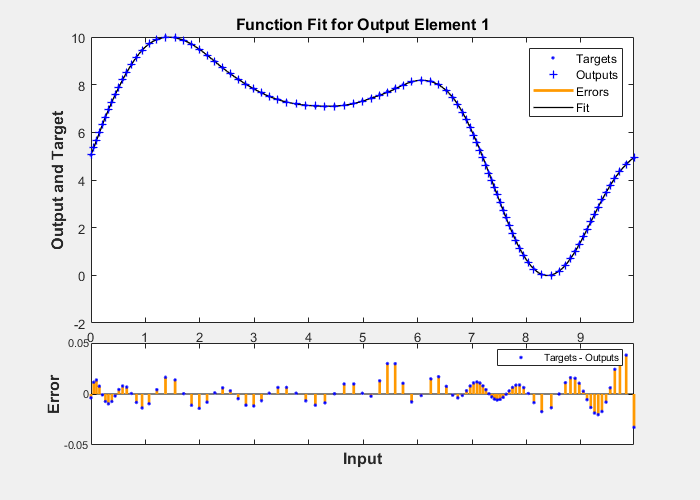# plotfit

Plot function fit

## Syntax

``plotfit(net,inputs,targets)``
``plotfit(net,inputs1,targets1,name1,inputs2,targets2,name2,...)``
``plotfit(...,'outputIndex',outputIndex)``

## Description

example

````plotfit(net,inputs,targets)` plots the output function of a network across the range of the inputs `inputs` and also plots target `targets` and output data points associated with values in `inputs`. Error bars show the difference between outputs and `targets`.The plot appears only for networks with one input.Only the first output/targets appear if the network has more than one output.```
````plotfit(net,inputs1,targets1,name1,inputs2,targets2,name2,...)` plots multiple sets of data.```
````plotfit(...,'outputIndex',outputIndex)` plots using an optional parameter that overrides the default index of the output element.```

## Examples

collapse all

This example shows how to use a feed-forward network to solve a simple fitting problem.

```[x,t] = simplefit_dataset; net = feedforwardnet(10); net = train(net,x,t);````plotfit(net,x,t)`## Input Arguments

collapse all

Input network, specified as a network object. To create a network object, use for example, `feedforwardnet` or `narxnet`.

Network inputs, specified as a matrix or cell array.

Network targets, specified as a matrix or cell array.

## Version History

Introduced in R2008a